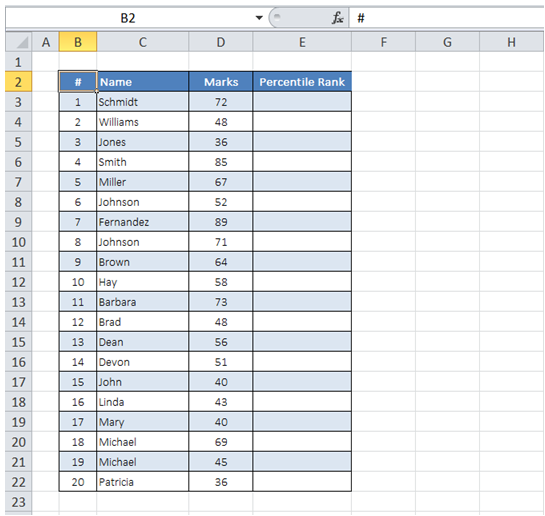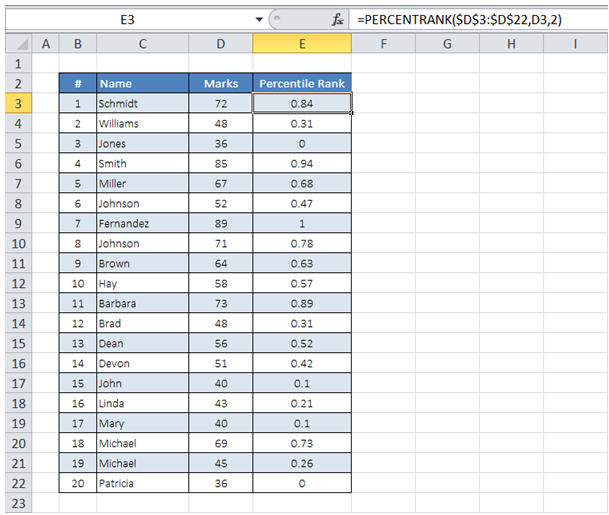## Get percentile rank for students in ExcelTo do it in Excel, here is the answer:

a) Enter the formula =PERCENTRANK(\$D\$3:\$D\$22,D3,2) in "Percentile Rank" column for first student. Drag the formula all the way down to end of column.

The first argument in formula corresponds to the array containing the scores, the second argument corresponds to individual Student score and the 3rd argument define the number of significant digits in the result.